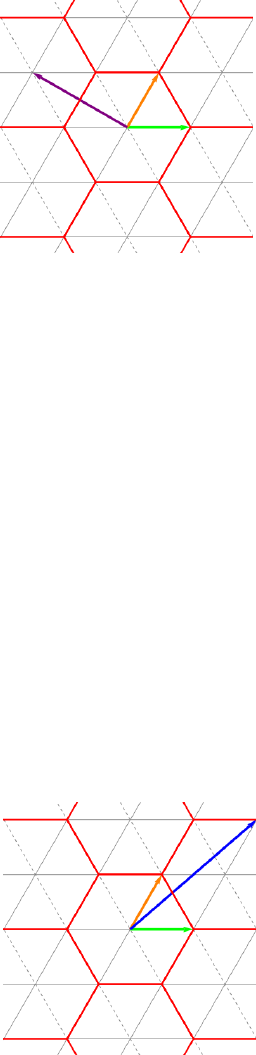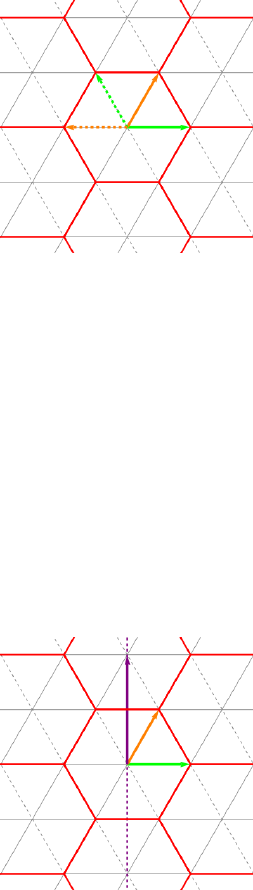Study Guides (400,000)
US (230,000)
UCLA (3,000)
MATH (300)
All (20)
Final

# MATH 33AH Lecture Notes - Lecture 3: Linear Map, Glossary Of Video Game Terms, Formula AtlanticExam

Department
Mathematics
Course Code
MATH 33AH
Professor
All
Study Guide
Final

This preview shows pages 1-2. to view the full 6 pages of the document.Math 33A Discussion Example
Austin Christian
October 23, 2016
Example 1. Consider tiling the plane by equilateral triangles, as below.
Let vand wbe the orange and green vectors in this ﬁgure, respectively, and let B=
{v,w}be the basis for R2formed by these two vectors. Let ube the purple vector.
(a) Sketch the vector 1
2B
in the ﬁgure.
(b) Give the components of uwith respect to the basis B.
(c) Let T:R2R2be the linear transformation that rotates the plane counterclockwise
through an angle of 2π/3. Find the matrix B[T]B.
(d) Is the terminal point of the vector 1008
2016 B
in the center of one of the red hexagons
or on an edge?
(Solution)
(a) Because this vector is given in the basis B={v,w}, the desired vector is equal to
1v+ 2w, which is the blue vector in the following ﬁgure:
1

Only pages 1-2 are available for preview. Some parts have been intentionally blurred.(b) We see that we can get to the terminal point of uby following wand then following
vtwice. Alternatively, we could follow vtwice and then follow w. In either case,
we see that
u=w2v=2
1B
.
(c) When we apply this rotation, vand ware carried to their dashed counterparts in the
following ﬁgure:
We see that T(v) = wvand T(w) = v, so
T1
0B=1
1B
and T0
1B=1
0B
.
This means that the matrix of Twith respect to Bis given by
B[T]B=11
1 0 .
(d) Notice that the vector 1
2B
lies in the center of a hexagon:
It is not diﬃcult to see that integer multiples of this vector will also lie in the centers
of various hexagons. Because our vector is such a multiple, it lies in the center of the
1008-th hexagon above the hexagon containing the origin.
2